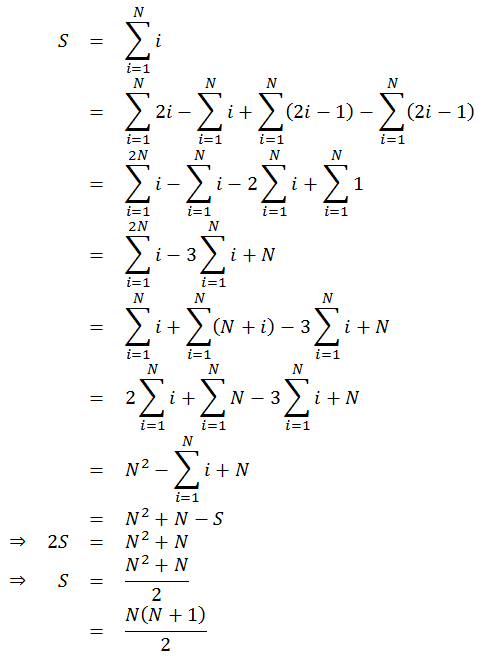# Sum of First N Numbers

by Zoran Horvat

## Problem Statement

Given a positive number N, write a function which returns sum of numbers 1 + 2 + ... + N.

Example: If N is 5, then return value should be 15 (1 + 2 + 3 + 4 + 5 = 15).

## Problem Analysis

This task can be solved quickly by iterating through the sequence:

``````function Sum(n)
begin
sum = 0
for i = 1 to n
sum = sum + i
return sum
end
``````

This function runs in time O(N) and space O(1). Now we can ask if there is a better solution in terms of running time. In order to make the function run in constant time, we need to entail a definite form of the sum. This is easy to do and here is one possible way:The function which calculates the sum of the sequence in O(1) time and O(1) space is then:
``````function Sum(n)
begin
return n * (n + 1) / 2
end
``````

## Implementation

Below is the console application in C# which lets the user enter N and then prints the sum of the sequence of N elements.

``````using System;

namespace SumOfSequence
{

public class Program
{

static int Sum(int n)
{
return n * (n + 1)  2;
}

static void Main(string[] args)
{

while (true)
{

Console.Write("Enter sequence length (zero to exit): ");

if (n <= 0)
break;

Console.WriteLine("Sum of the sequence is {0}\n", Sum(n));

}

}

}
}
``````

## Demonstration

Here is the possible output of the application listed above:

```            ```
Enter sequence length (zero to exit): 4
Sum of the sequence is 10

Enter sequence length (zero to exit): 5
Sum of the sequence is 15

Enter sequence length (zero to exit): 43127
Sum of the sequence is 929990628

Enter sequence length (zero to exit): 0
```
```